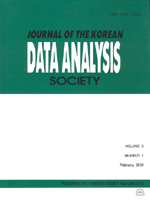상세검색
최근 검색어 전체 삭제
다국어입력
즐겨찾기0KCI등재 학술저널

# The Modified Confidence Intervals by Optimal Conditions in One-Factor Component-of-Variance Model

• 등재여부 : KCI등재
• 2006.06
• 881 - 894 (14 pages)

The confidence intervals for sigma_A ^2 are given in various forms by many authors in one-factor component-of-variance model y_ij =mu + A_i +e_ij In this model, Graybill and Wang s(1980) confidence intervals by using Satterthwaite s(1946) method for sigma_A ^2 are given by an asymptotic confidence intervals. The simulations results of confidence coefficients are not fit exactly for the given confidence coefficient. Thus, we would like to give one of the optimal conditions and we can find out the better confidence intervals by the optimal conditions for sigma_A ^2 or the given Graybill and Wang s(1980) confidence intervals using Satterthwaite s(1946) method in one-factor components-of-variance model.

1. Introduction

2. One-Factor Components-of-Variance Model

3. Modified Confidence Intervals

4. Simulation Results

References